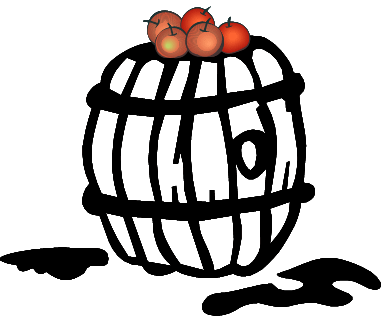Repeated Inspections

A widespread misconception is that if one inspection is good, repeated inspections must be better.

It is not uncommon to hear of great (calculated) “improvements” in POD (probability-of-detection) through repeated inspections. The “logic” of the purported improvement is something like this:

If $$POD_{single \, inspection} = 0.9$$, then $$POM$$ (probability of miss) = $$1-POD = 0.1$$.

The probability of missing something twice is $$POM_2$$. Therefore $POD_{double \, inspection} = 1-POM_2 = 1-0.01 = 0.99$

Thus the redundant inspection has 99% POD compared with the original, single inspection, POD of 90%

All this seems quite reasonable. What could possibly be wrong?

The problem (again) is the statistical fine-print. The trouble lies in this statement:

The probability of missing something twice is POM2.

This statement is sometimes right – often times wrong. Implicit (and thus overlooked, or worse, ignored) is that the inspections must be independent, and looking at one thing twice is hardly the same as looking at two independent things.

Red Apples and Green Apples

Let’s try this thought experiment: We have a barrel of red and green apples and we want to know the fraction of each color.I take an apple, examine it, and pronounce it to be red. I give the same apple to Thomas, who concurs that it is red, and passes the apple to Richard, who also agrees that the apple is red. We have three opinions on the color of one apple. How much more do we know of the fraction of red apples in the barrel than we knew after the first examination?

Now Richard gives the apple to Harold, who says that it is green, not red.

Now, how much more do we know of the fraction of red apples in the barrel than we knew after the first examination?